Do you like the article?
Share it with others -
Use new possibilities of MetaTrader 5

# Expert System 'Commentator'. Practical Use of Embedded Indicators in an MQL4 Program

16 April 2007, 16:111
3 956

An experienced trader will never open a position if no technical indicator confirms this decision. For example, the trader has conducted technical analyses and concluded that it is possible to open a long position for a security, let it be EURUSD. To enter the market at the current price (see Fig. 1 below), an experienced trader checks the results obtained by an indicator, for example, Stochastic Oscillator, and waits until it gives a confirming signal. The indicator and its signals are described here: https://www.metatrader5.com/en/terminal/help/indicators/oscillators/so. In our exemplary case, let us take a signal that is described there.

"...Buy when the %K line rises above the %D line and sell when the %K line falls below the %D line."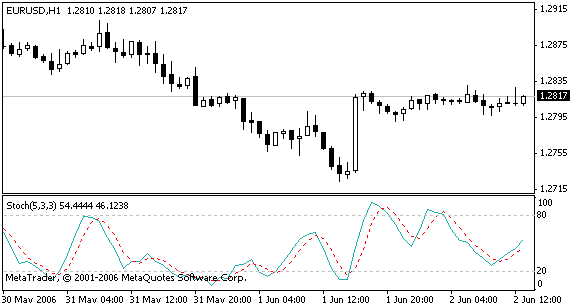Fig. 1 Prices for EURUSD

%K is the main line, %D (dashed) is the signal line. MQL4 allows use of embedded functions for calculations of indicators. The full list of those functions is given at the end of this article and in the MQL4 documantation. Indicator Stochastic Oscillator is among them, too. To calculate values of the main and of the signal line, the function below is used:

```double iStochastic( string symbol, int timeframe, int %Kperiod,
int %Dperiod, int slowing, int method,
int price_field, int mode, int shift)```

The function is described in more details in the MQL4 documentation.

Below is the value calculation for the main line of indicator Stochastic Oscillator in MQL4:

`iStochastic(NULL,0,5,3,3,MODE_SMA,0,MODE_MAIN, i)`

Parameters 3,5,5 here are period values of the indicator averages, MODE_SMA is the method to calculate moving average, i – the number of the candlestick, for which the value is calculated, parameter MODE_MAIN informs that the main line (%K) must be calculated. Respectively, below is the calculation of the signal line:

`iStochastic(NULL,0,5,3,3,MODE_SMA,0,MODE_SIGNAL, i)`

When using the embedded functions of indicator calculation, it is necessary to specify the chart timeframe and symbol. In our case, the current symbol and the current timeframe of the chart (parameters NULL and 0) are considered. It is necessary to remember that calculations can be made on any symbols and for any timeframes. It can be seen in the Stochastic Oscillator chart that the main line value is equal to 54.4444 and the signal line value is 46.1238, i.e.:

```iStochastic(NULL,0,5,3,3,MODE_SMA,0,MODE_MAIN, i) >
iStochastic(NULL,0,5,3,3,MODE_SMA,0,MODE_SIGNAL, i)```

It will be easy for us now to automate indicator calculations. It would be convenient if the indicator did not consume space in the price chart. The best soultion would be to eliminate the indicator graph from the chart and only show the signal calculated above. The most popular method to show signals is placing Wingdings characters in the chart. We will discuss this method later and now let us try to use another feature of MQL4. It is operator named Comment().

`void Comment( ...)`

It helps to show any comments in the chart price.

```//STOCH

double valSTOCH=0;
if(iStochastic(NULL,0,5,3,3,MODE_SMA,0,MODE_MAIN,0)
>iStochastic(NULL,0,5,3,3,MODE_SMA,0,MODE_SIGNAL,0))

if(iStochastic(NULL,0,5,3,3,MODE_SMA,0,MODE_MAIN,0)
<iStochastic(NULL,0,5,3,3,MODE_SMA,0,MODE_SIGNAL,0))

The source code above translates the signal into a text in order to show it in the chart.

`Comment(commentSTOCH + "\n");`

The Comment is convenient since the user can utilize the line-feed character to visualize comments line by line. Using the code above, a trader can eliminate the graph of Stochastic Oscillator. It will have an alarm system that will show the current market developments as texts.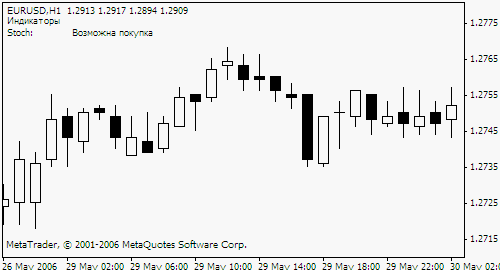Now let's consider, for instance, Commodity Channel Index (CCI).

"...measures the deviation of the commodity price from its average statistical price. High values of the index point out that the price is unusually high being compared with the average one, and low values show that the price is too low. In spite of its name, the Commodity Channel Index can be applied for any financial instrument, and not only for the wares."

Having automated CCI signal calculations, we will get an additional comment like "The price is presumably too high". Using, for example, technical indicator named Acceleration/Deceleration (AC) that measures acceleration/deceleration of the current trend, the trader can be informed even better. "...If you realize that Acceleration/Deceleration is a signal of an earlier warning, it gives you evident advantages."

This is how the code displaying signals from several indicators may look:

```//   Demarker
double valDem=iDeMarker(NULL, 0, 13, 0);
string commentDem = "DeMarker:    ";
string commentDemAdd = "   No data";

if (valDem < 0.30)
commentDemAdd =  "   Prices are expected to go Up";

if (valDem > 0.70)
commentDemAdd =   "   Prices are expected to go Down";

//ATR
double valATR=iATR(NULL, 0, 12, 0);
string commentATR = "ATR:           ";
commentATR=commentATR + "   Trend will probably change " + valATR;

//CCI
double valCCI=iCCI(NULL,0,12,PRICE_MEDIAN,0);
string commentCCI = "CCI:            ";
string commentCCIAdd = "   No data ";
if (valCCI > 100)
commentCCIAdd =  "   Overbought " +
"(a correcting down-trend is possible) ";

if (valCCI < -100)
commentCCIAdd =  "   Oversold " +
"(a correcting up-trend is possible) ";

commentCCI =  commentCCI + commentCCIAdd + valCCI;

//MFI
double valMFI=iMFI(NULL,0,14,0);
string commentMFI = "MFI:            ";
string commentMFIAdd = "   No data ";
if (valMFI > 80)
commentMFIAdd =  "    a possible market peak ";

if (valMFI < 20)
commentMFIAdd =  "    a possible market trough ";

commentMFI =  commentMFI + commentMFIAdd + valMFI;```

Calculations can also be made with arrays. Functions that end with OnArray can be used for this purpose, for example, function iMAOnArray that calculates moving average on the data array. It is interesting that some indicators sometimes tend to give opposite signals. Well, for a trader, no signal is a signal, too. Trading is not only making a decision about the direction to open a position, but also the open time. Expert system "Commentator" calculates signals for only the current timeframe, though a trader can add calculations for larger or smaller timeframes to the program code. It should also be noted that the use of MQL4 does not tighten the trader in any way, but elevates the mind and enhances functionality.

The functions of embedded indicator calculations available in the MQL4 are given below:

```iAC
iAlligator
iATR
iAO
iBearsPower
iBands
iBandsOnArray
iBullsPower
iCCI
iCCIOnArray
iCustom
iDeMarker
iEnvelopes
iEnvelopesOnArray
iForce
iFractals
iGator
iIchimoku
iBWMFI
iMomentum
iMomentumOnArray
iMFI
iMA
iMAOnArray
iOsMA
iMACD
iOBV
iSAR
iRSI
iRSIOnArray
iRVI
iStdDev
iStdDevOnArray
iStochastic
iWPR```

Translated from Russian by MetaQuotes Software Corp.
Original article: https://www.mql5.com/ru/articles/1406

Attached files |
Commentator.mq4 (7.03 KB)

#### Other articles by this author

Last comments | Go to discussion (1)[Deleted] | 27 Apr 2007 at 14:27
Hm .. nice but

Comment() is using proportional port so if you tries to formatoutput like:

`P1=10;T1=2;QQQ="Price:                 "+P1;WWW="Times:                 "+T1;Comment(QQQ+"\n"+WWW);`
You get this:

`Price:                 "+P1;Times:                 "+T1;`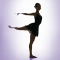Pivot Points Helping to Define Market Trends
Pivot point is a line in the price chart that shows the further trend of a currency pair. If the price is above this line, it tends to grow. If the price is below this line, accordingly, it tends to fall.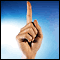Events in МetaТrader 4
The article deals with programmed tracking of events in the МetaТrader 4 Client Terminal, such as opening/closing/modifying orders, and is targeted at a user who has basic skills in working with the terminal and in programming in MQL 4.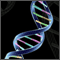Genetic Algorithms vs. Simple Search in the MetaTrader 4 Optimizer
The article compares the time and results of Expert Advisors' optimization using genetic algorithms and those obtained by simple search.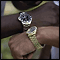Synchronization of Expert Advisors, Scripts and Indicators
The article considers the necessity and general principles of developing a bundled program that would contain both an Expert Advisor, a script and an indicator.